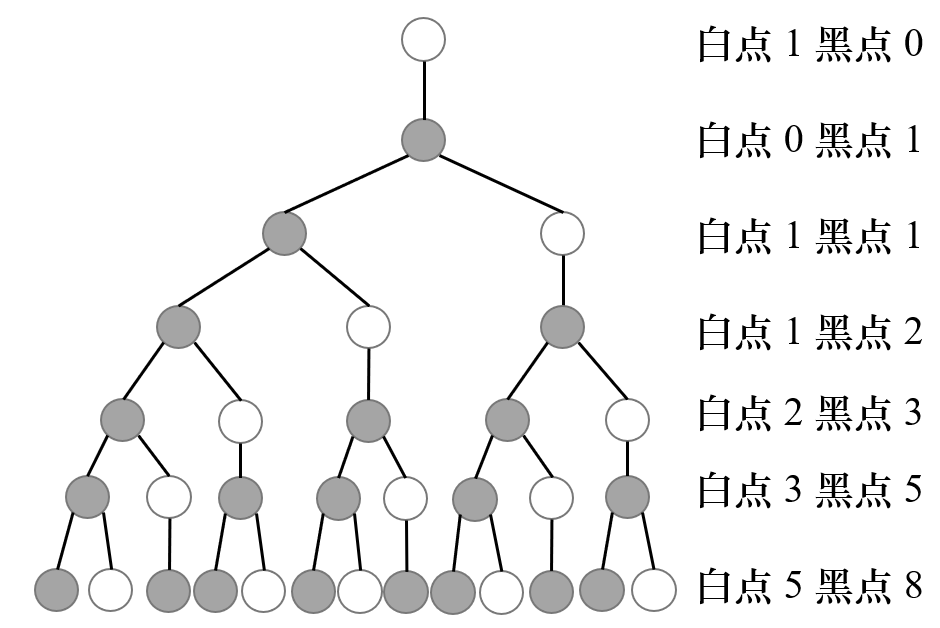# 幻魔皇 题解【数列】【递推】【斐波那契树】

这个题暴力分倒是挺多的……正解也不是很难想

## 输入输出样例

 raviel.in raviel.out 5 0 2 3 3 1 1 0 0 0 0

## 题解：

感觉斐波那契树是一个挺有意思的东西。因为每一层的黑点数和白点数都是上面两层相应点数的和，所以会有很多递推性质。一开始打了个$O(n^3)$的暴力，可以拿$80$分，因为对于同一层的同色节点，它们的状态和子树都是一样的，因此只需要计算一次，剩下的直接统计答案就可以了。这里的思路类似于记忆化搜索，如果某一深度某种颜色的点被找到了，那么可以直接把之前统计的子树的贡献加上就可以了。

这个思路是在使树不动，节点动，这样就要每层都做一遍，并合并左右子树，我们可不可以让树动，节点不动呢？我们如果只枚举两棵子树的深度，时间复杂度就是$O(n^2)$，希望能在枚举深度的过程中顾虑到所有节点的情况。

我们可以枚举白点和黑点做子问题，对于白点，它对下方能做的贡献就是与子树中所有的白点组成一对，此时只需要枚举每个深度有多少个白点就可以了。为了方便说明，我们把当前白点命名为点①，下面那个白点命名为点②。如果要统计距离某个①深度为$i$的白点②个数就是$Fib_i$（$Fib$数组的定义为$Fib_0=1,Fib_1=0$然后递推），但是①可能有很多个，我们还需要计算合法的①的个数。

合法的①必须有深度为$i$的后代，否则会得到深度超过$n$的位置，导致不合法的状态。我们还需要预处理出$Fib$数组的前缀和$sum$，这样可以直接利用$sum$，求出深度$\le n-i$的白点①个数，使答案加上$sum_{n-i}\times Fib_i$。

对于黑点，称之为❶，它可以作为LCA连接下方的两个白点①和②，此时我们枚举左右孩子的深度，然后把它们拼起来，这时❶的合法个数也是根据①和②来确定的。此时不能直接对❶的后代进行枚举，因为❶有两棵子树，这样的话①②可能在同一棵子树中。这时考虑对左儿子$Fib_{i-1}$和右儿子的$Fib_{j-1}$相乘对答案做出贡献即可。合法的❶种类有$Fib_{n-\max(i,j)}$种。

因为我们要枚举$(i,j)$，所以时间复杂度是$O(n^2)$。

## Code：

#include<cstdio>
long long Max(long long x,long long y)
{
return x>y?x:y;
}
long long p=123456789;
long long f,sum;
long long ans;
int main()
{
int n;
scanf("%d",&n);
f=1;
sum=sum=1;
for(int i=2;i<=n;++i)
{
f[i]=(f[i-1]+f[i-2])%p;
sum[i]=(sum[i-1]+f[i])%p;
}

for(int i=1;i<n;++i)//距离某白点为i
{
ans[i]=(ans[i]+sum[n-i-1]*f[i])%p;//这里是计算当前层为白点时的贡献
//             sum有多少个这样的点;f每个点的贡献
for(int j=1;j<n;++j)//距离另一白点为j
if(n-Max(i+1,j))
ans[i+j]=(ans[i+j]+(sum[n-Max(i,j)]-1)*f[i]%p*f[j-1]%p)%p;
//                 有多少个这样的点 黑儿子的贡献 白儿子的贡献
}
for(int i=1;i<=2*n;++i)
printf("%lld ",ans[i]);
return 0;
}

### 说点什么Subscribe

/* */